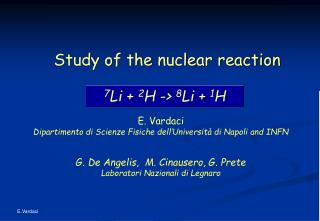DownloadDownload Presentation7 Li + 2 H -> 8 Li + 1 H

# 7 Li + 2 H -> 8 Li + 1 H

Télécharger la présentation## 7 Li + 2 H -> 8 Li + 1 H

- - - - - - - - - - - - - - - - - - - - - - - - - - - E N D - - - - - - - - - - - - - - - - - - - - - - - - - - -
##### Presentation Transcript

1. Study of the nuclear reaction 7Li + 2H -> 8Li + 1H E. Vardaci Dipartimento di Scienze Fisiche dell’Università di Napoli and INFN G. De Angelis, M. Cinausero, G. Prete Laboratori Nazionali di Legnaro

2. 7Li + 2H -> 8Li + 1H Elab = 25MeV C. Rubbia et al., NIM A568 (2006) 475

3. Questions • What is the angular distribution of 8Li • How big is the cross section for the 8Li production

4. 8Li 7Li Two possible mechanisms Formation and decay of a compound nucleus 7Li + 2H -> 9Be* -> 8Li + 1H Neutron Pick-up 2H 7Li

5. Two Body Kinematics 3 Entrance channel CN Exit channel 2 1 Q = E3 + E4 – E1 4 E1 + Q = E3 + E4 Qgg = M1 + M2 – M3 – M4 Qfu = M1 + M2 – MCN ELab = T0 + TCM

6. TRIGGERS Fission Fragments in ring E/F/G CORSET (E. Kozulin group, FLNR) Evaporation Residues (4 PPAC- PPAC) The 8pLP setup MAX ENERGYWall: up to 64 AMeV Ball : up to 34 AMeV ENERGY THRESHOLDS0.5 AMeV for p and a 2-3 AMeV for 12C

7. Er DE E- DE Technique

8. Er DE PSD Technique Used for particles that stop in the first stage of the telescope.

9. 7Li + 2H -> 8Li + 1H

10. 7Li + 2H -> 8Li + 1H

11. 7Li + 2H -> 9Be* -> 8Li + 1H E * = To + Qfu 5.364 T0 5555 -190.749 8Li + 1H 7Li + 2H Qgg = -190.749 22250.7 16886.469 16695.72 Elab = 25MeV

12. Population of 8Li 9Be* 5364 Sn

13. Hydrogen pollution in the Target

14. E-DE Particle Identification DET #72 20.6°

15. E-DE Particle Identification DET #99 22.2°

16. Protons Energy Spectra DET #72 20.6°

17. Protons Energy Spectra DET #308 43.2°

18. 7Li + 2H -> 1H (Energy)

19. Furthermore • Normalize X-Sections • Energy Checks for the Excited states • Convert to CM frame • Analyze t and a channels

20. 7Li + 2H -> 1H (Energy)

21. 7Li + 2H -> 1H (Energy)

22. 7Li + 2H -> 1H (Energy)

23. 7Li + 2H -> 1H (Energy)

24. Pollution 2+ (g.s.) 3+ (2nd) 1+ (3rd) 1+ (1st)

25. 7Li + 2H -> 1H Lab Angular Distribution for E1 Preliminary

26. 2H(7Li,p) 8Li CM Angular Distribution for E1 8 MeV 12 MeV

27. 7Li + 2H -> 1H Lab Angular Distribution for E2

28. 7Li + 2H -> 1H Lab Angular Distribution for E3

29. 7Li + 2H -> 1H Lab Angular Distribution for E4

30. 7Li + 2H -> 8Li + 1H

31. 7Li (d, p) 8Li 7Li (d, 3He) 6He 7Li (d, t) 6Li More Channels 7Li + 2H